MCQs from NCERT Exemplar

Chapter 1 Class 10 Real Numbers
Serial order wise

## (c) an odd integer   (d) an even integer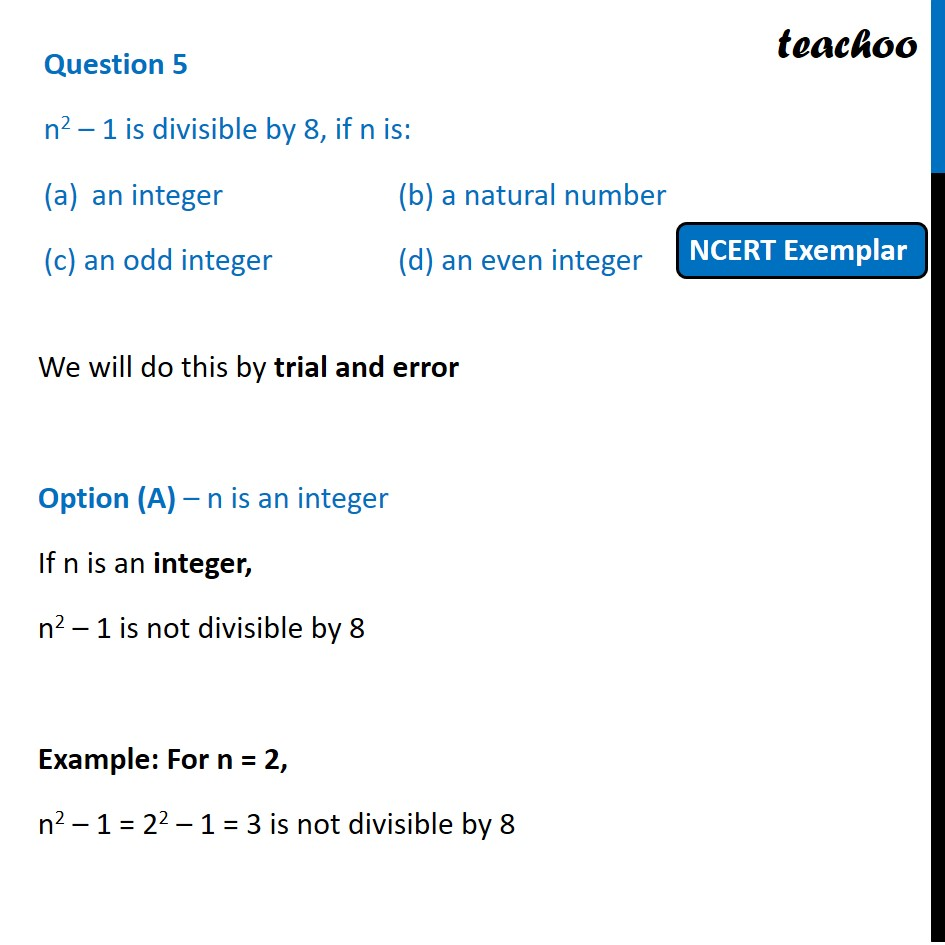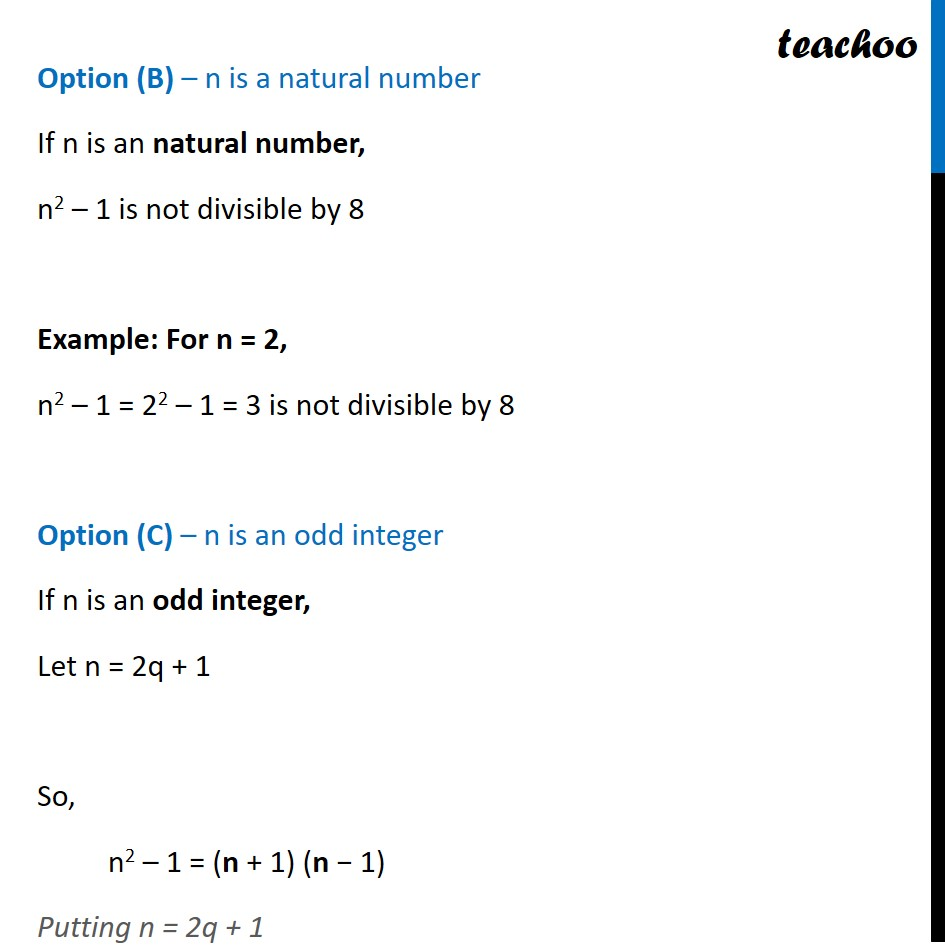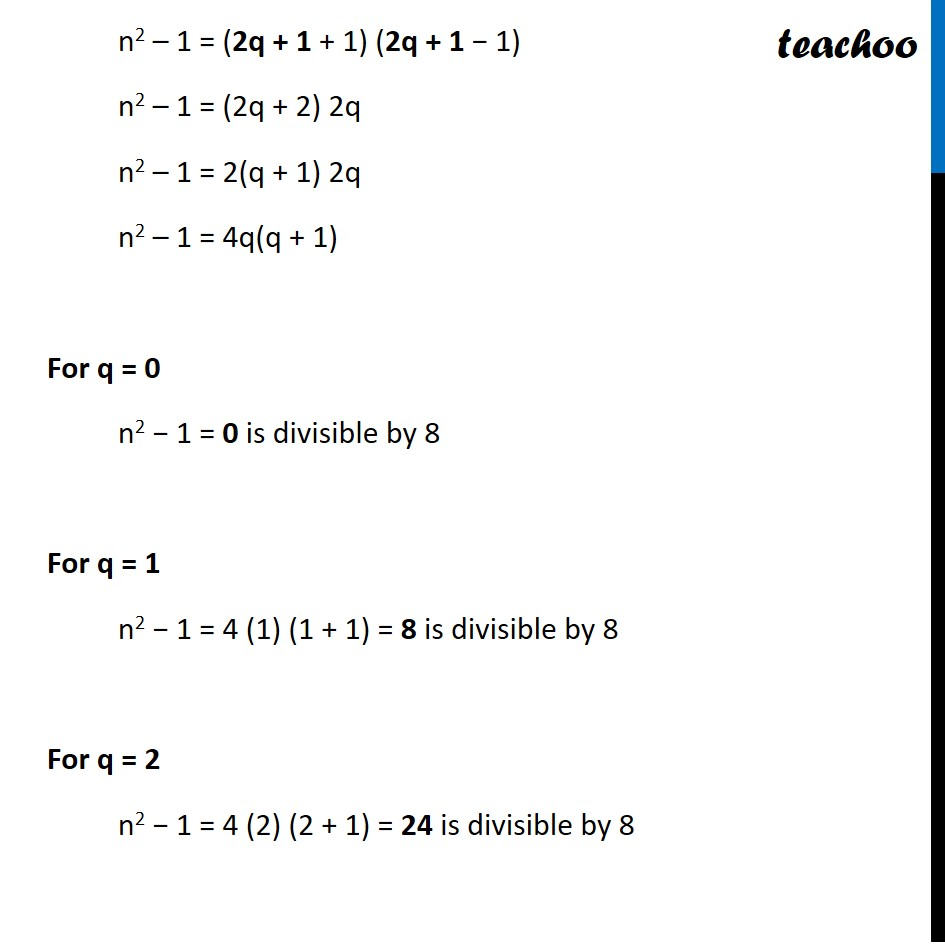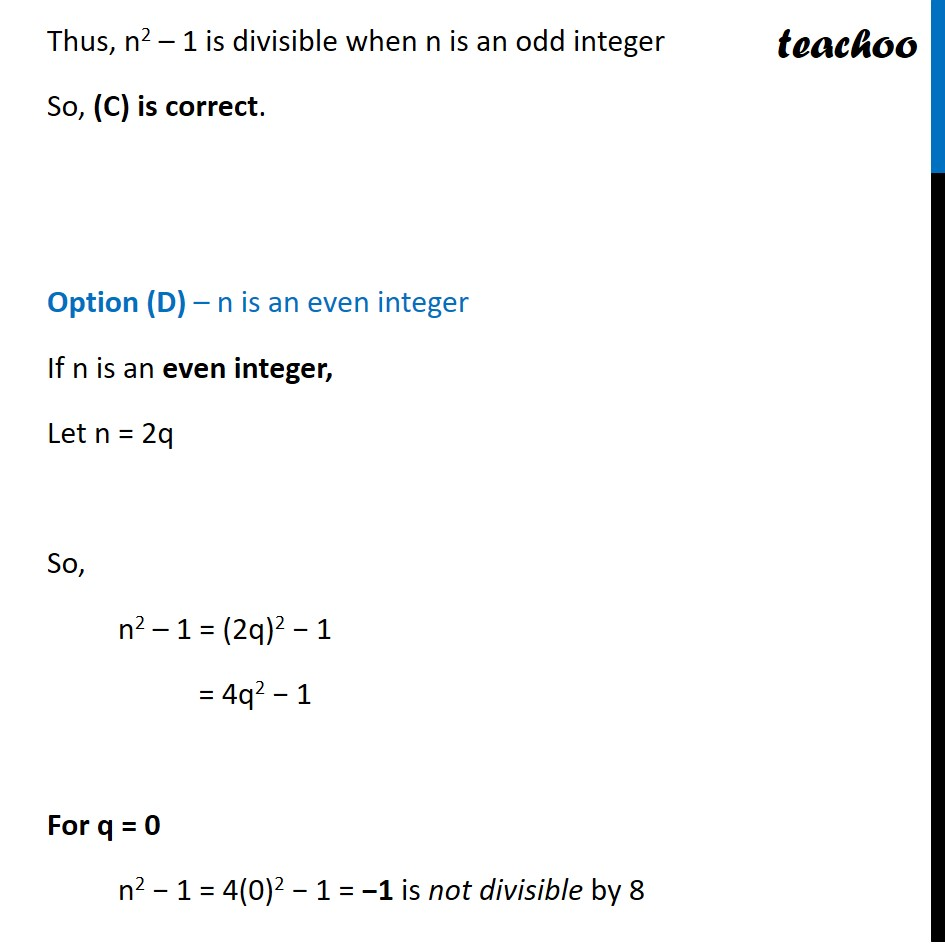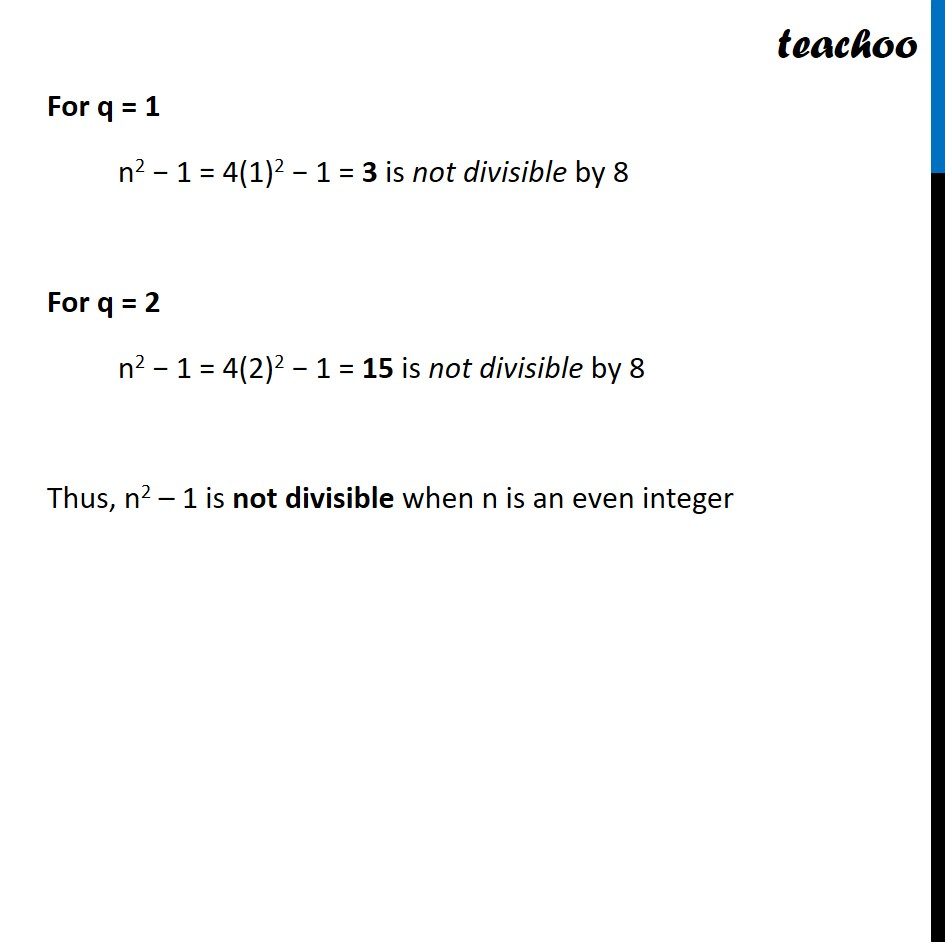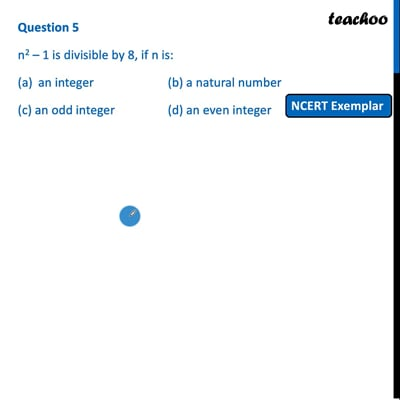This video is only available for Teachoo black users

Introducing your new favourite teacher - Teachoo Black, at only ₹83 per month

### Transcript

Question 5 n2 – 1 is divisible by 8, if n is: an integer (b) a natural number (c) an odd integer (d) an even integer We will do this by trial and error Option (A) – n is an integer If n is an integer, n2 – 1 is not divisible by 8 Example: For n = 2, n2 – 1 = 22 – 1 = 3 is not divisible by 8 Option (B) – n is a natural number If n is an natural number, n2 – 1 is not divisible by 8 Example: For n = 2, n2 – 1 = 22 – 1 = 3 is not divisible by 8 Option (C) – n is an odd integer If n is an odd integer, Let n = 2q + 1 So, n2 – 1 = (n + 1) (n − 1) Putting n = 2q + 1 n2 – 1 = (2q + 1 + 1) (2q + 1 − 1) n2 – 1 = (2q + 2) 2q n2 – 1 = 2(q + 1) 2q n2 – 1 = 4q(q + 1) For q = 0 n2 − 1 = 0 is divisible by 8 For q = 1 n2 − 1 = 4 (1) (1 + 1) = 8 is divisible by 8 For q = 2 n2 − 1 = 4 (2) (2 + 1) = 24 is divisible by 8 Thus, n2 – 1 is divisible when n is an odd integer So, (C) is correct. Option (D) – n is an even integer If n is an even integer, Let n = 2q So, n2 – 1 = (2q)2 − 1 = 4q2 − 1 For q = 0 n2 − 1 = 4(0)2 − 1 = −1 is not divisible by 8 For q = 1 n2 − 1 = 4(1)2 − 1 = 3 is not divisible by 8 For q = 2 n2 − 1 = 4(2)2 − 1 = 15 is not divisible by 8 Thus, n2 – 1 is not divisible when n is an even integer